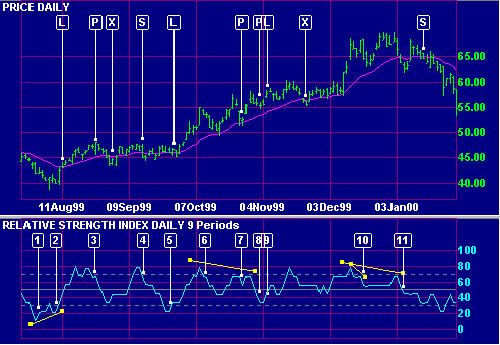# Relative Strength Index (RSI) Indicator

The RSI Indicator is a popular momentum oscillator developed by J. Welles Wilder Jr. and detailed in his book New Concepts in Technical Trading Systems.

It compares upward movements in closing price to downward movements over a selected period. Wilder originally used a 14 day period, but 7 and 9 days are commonly used to trade the short cycle and 21 or 25 days for the intermediate cycle. Please note that Wilder does not use the standard moving average formula and the time period may need adjustment.

The Relative Strength Index is smoother than the Momentum or Rate of Change oscillators and is not as susceptible to distortion from unusually high or low prices at the start of the window (detailed in Momentum). It is also formulated to fluctuate between 0 and 100, enabling fixed Overbought and Oversold levels.

Different signals are used in trending and ranging markets. The most important signals are taken from overbought and oversold levels, divergences and failure swings.

### Ranging Markets

Set the Overbought level at 70 and Oversold at 30.

• Go long when RSI falls below the 30 level and rises back above it
or on a bullish divergence where the first trough is below 30.
• Go short when RSI rises above the 70 level and falls back below it
or on a bearish divergence where the first peak is above 70.

Failure swings strengthen other signals.

### Trending Markets

Only take signals in the direction of the trend.

• Go long, in an up-trend, when RSI falls below 40 and rises back above it.
• Go short, in a down-trend, when RSI rises above 60 and falls back below it.

Exit using a trend indicator.

Take profits on divergences. Unless confirmed by a trend indicator, Relative Strength Index divergences are not strong enough signals to trade in a trending market.

### Example

Wal-Mart Stores Inc. is plotted with a   21 day exponential moving average (MA) and    9 day Relative Strength Index. A 2-day closing filter is used with the MA.Mouse over chart captions to display trading signals.

1. Price is trending downwards (staying below the moving average). Do not take long signals until the MA turns upward, otherwise we are trading against the trend.
2. A bullish divergence on Relative Strength Index is reinforced by completion of a failure swing at . Go long [L] when the MA slopes upwards and RSI crosses to above 40.
3. RSI completes a minor failure swing at . Take profits [P] and exit the remaining position [X] when there are two closes below the MA.
Do not go short as price is trending upwards (staying above the moving average).
4. Price has started to fluctuate around the moving average, signaling a ranging market. Go short [S] when RSI crosses from above to below 70.
5. Go long [L] when RSI crosses from below to above 30.
6. There has been a breakout from the trading range and price is trending upwards. Do not close the long position.
7. Take profits [P] on the bearish divergence (Price has completed a higher peak while RSI has experienced a lower peak).
8. Take profits [P]. A bearish triple divergence is confirmed by completion of a large failure swing at .
9. Increase your long position [L]. RSI has crossed from below to above 40 during an up-trend.
Exit your position [X] when there are two closes below the MA. Do not go short as the MA still slopes upwards.
10. A small bearish divergence warns of a possible trend reversal.
11. A bearish triple divergence is reinforced by completion of a failure swing at . Wait until the MA has turned down and RSI has crossed to below 60 before entering a short trade [S].

### Setup

See Indicator Panel for directions on how to set up Relative Strength Index.

The default RSI window is set at 14 days with Overbought/Oversold levels at 70% and 30%. To alter the default settings - see Edit Indicator Settings.

## Relative Strength Index Formula

The steps in calculation of the Relative Strength Index are:

1. Decide on the RSI Period, based on the time frame that you wish to analyze.
2. Compare Closing price [today] to Closing price [yesterday].
3. For the RSI Period, add all upward movements in Closing price.
4. For the RSI Period, add all downward movements in Closing price.
5. Calculate the exponential moving average* of price movements:

Average Upward Price Move = Exponential Moving Average of Upward Movements
Average Downward Price Move = Exponential Moving Average of Downward Movements

6. Calculate Relative Strength (RS):

RS = Average Upward Price Move / Average Downward Price Move

7. Calculate the Relative Strength Index:

RSI = 100 - 100 / ( 1 + RS )

### *Welles Wilder's Indicators

Users should beware, when setting time periods for Welles Wilder's indicators, that he does not use the standard exponential moving average formula.

See Wilder Moving Average

We recommend that users try shorter time periods when using one of the above indicators. For example, if you are tracking a 30-day cycle you would normally select a 15-day Indicator Time Period. With the RSI, adjust the time period as follows:

RSI time period = (n + 1) / 2       = (15 + 1) / 2       = 8 days